### Home > MC2 > Chapter 2 > Lesson 2.3.1 > Problem2-97

2-97.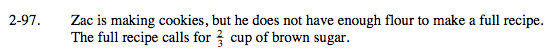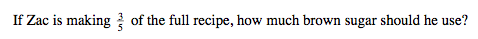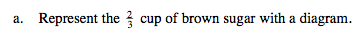First draw a rectangle to represent a full cup of brown sugar. Now try to solve the problem with this information.

$\frac{2}{3} \text{ is the same thing as 2 one-thirds.}$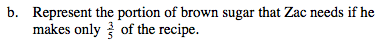How can you subdivide your shaded diagram from part (a) into fifths (5 equal parts)?

Which part of the sugar is 3 of the fifths?

One way this can be represented is...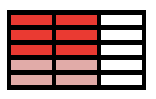Label the portion of brown sugar Zac needs.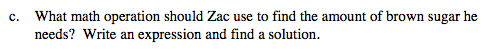$\text{What fraction of a cup will Zac use? What operation with } \frac{2}{3} \text{ and } \frac{3}{5} \text{ will get that answer?}$